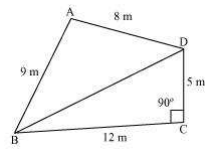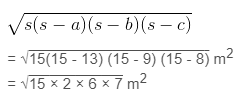Guru

# A park, in the shape of a quadrilateral ABCD, has C = 90°, AB = 9 m, BC = 12 m, CD = 5 m and AD = 8 m. How much area does it occupy? Q.1

• 0

What is the best way for solving the question from class 9th ncert math of Heron’s Formula chapter of Ncert of exercise 12.2  of math. What is the best way for solving this question please guide me the best way for solving this question A park, in the shape of a quadrilateral ABCD, has C = 90°, AB = 9 m, BC = 12 m, CD = 5 m and AD = 8 m. How much area does it occupy?

Share

1. First, construct a quadrilateral ABCD and join BD.

We know that

C = 90°, AB = 9 m, BC = 12 m, CD = 5 m and AD = 8 m

The diagram is:Now, apply Pythagoras theorem in ΔBCD

BD2 = BC2 +CD2

⇒ BD2 = 122+52

⇒ BD2 = 169

⇒ BD = 13 m

Now, the area of ΔBCD = (½ ×12×5) = 30 m2

The semi perimeter of ΔABD

(s) = (perimeter/2)

= (8+9+13)/2 m

= 30/2 m = 15 m

Using Heron’s formula,

Area of ΔABD= 6√35 m2 = 35.5 m2 (approximately)

∴ The area of quadrilateral ABCD = Area of ΔBCD+Area of ΔABD

= 30 m2+35.5m2 = 65.5 m2

• 0